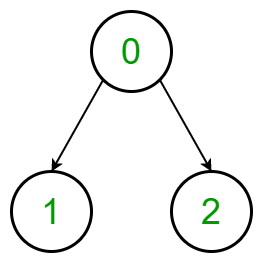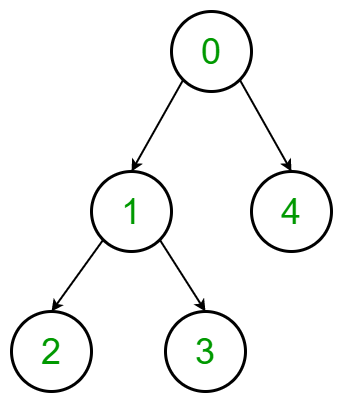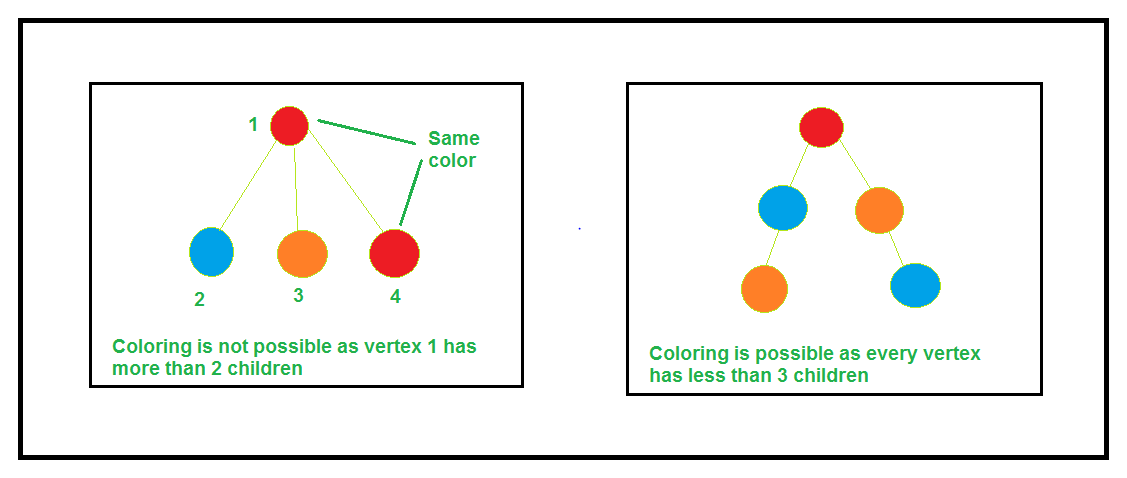# Minimum cost to colour a Tree with no 3 adjacent vertices having same colour

• Difficulty Level : Hard
• Last Updated : 21 Jun, 2021

Given a tree with N nodes value from 0 to (N – 1) and a 2D array arr[][] of size dimensions 3xN, where arr[i][j] denotes the cost of coloring jth nodes with color value i. The task is to find the minimum cost of coloring the node of the given tree such that every path of length 3 is colored with distinct colors. If there is any possible way of colouring the nodes of the tree then print the cost else print “Not Possible”.

Examples:

Attention reader! Don’t stop learning now. Get hold of all the important DSA concepts with the DSA Self Paced Course at a student-friendly price and become industry ready.  To complete your preparation from learning a language to DS Algo and many more,  please refer Complete Interview Preparation Course.

In case you wish to attend live classes with experts, please refer DSA Live Classes for Working Professionals and Competitive Programming Live for Students.

Input: arr[][] = {{3, 2, 3}, {4, 3, 2}, {3, 1, 3}},
Tree:Output:
Explanation:
Color the vertex 0 with type 1 color, cost = 3
Color the vertex 1 with type 3 color, cost = 1
Color the vertex 2 with type 2 color, cost = 2
Therefore the minimum cost is 3 + 1 + 2 = 6

Input: arr[][] = {{3, 4, 2, 1, 2}, {4, 2, 1, 5, 4}, {5, 3, 2, 1, 1}},
Tree:Output: NOT POSSIBLE

Approach: The idea is to make an observation. We need to observe that the answer is not possible if there is a vertex that has more than two edges. The answer exists only for a chain structure, i.e.,• Initially, we check if, for any node, there exist more than two children or not.
• If there exist, then the answer is not possible.
• If there doesn’t exist, then there are only 3P2 available permutations. So, simply check for all the six possible permutations and find the minimum cost.

Below is the implementation of the above approach:

## C++

 `// C++ program to find the``// minimum possible cost``// to colour a given tree` `#include ``using` `namespace` `std;` `// Class to define a tree``class` `tree {``    ``vector > g;``    ``vector<``int``> chain;``    ``int` `minimum;` `public``:``    ``// Constructor``    ``tree(``int` `n)``    ``{``        ``g = vector >(n);``        ``minimum = 1e6;``    ``}` `    ``// Function for pushing edges``    ``void` `addEdge(``int` `u, ``int` `v)``    ``{``        ``g[v].push_back(u);``        ``g[u].push_back(v);``    ``}` `    ``// Dfs function to make the chain``    ``// structure of tree in a vector``    ``void` `dfs(``int` `v, ``int` `p = -1)``    ``{``        ``chain.push_back(v);` `        ``for` `(``auto` `i : g[v]) {``            ``if` `(i == p)``                ``continue``;` `            ``dfs(i, v);``        ``}``    ``}` `    ``// Function that checks all the``    ``// six different type of``    ``// coloring and find the``    ``// minimum of them``    ``void` `check(``int` `n, ``int` `a, ``int` `b,``               ``vector > cost)``    ``{``        ``int` `sum = 0;``        ``vector<``int``> res(n);` `        ``// Assign the color type 1``        ``// to the first element``        ``res = a;` `        ``// Assign the color type 2``        ``// to the second element``        ``res = b;` `        ``// Add the cost of the color``        ``// of the first element``        ``sum += cost[a][chain];` `        ``// Add the cost of the color``        ``// of the second element``        ``sum += cost[b][chain];` `        ``for` `(``int` `i = 2; i < n; i++) {` `            ``// Assign the next element in chain``            ``// with different color``            ``res[i] = 3 - res[i - 1] - res[i - 2];` `            ``// Add the cost of the element color``            ``sum += cost[res[i]][chain[i]];``        ``}` `        ``// Finding the minimum from all cases``        ``if` `(sum < minimum)``            ``minimum = sum;``    ``}` `    ``// Function to find the``    ``// minimum possible cost``    ``// to colour a given tree``    ``void` `minimumCost(``int` `n,``                     ``vector > cost)``    ``{``        ``for` `(``int` `i = 0; i < n; i++) {` `            ``// Condition to check if``            ``// any vertex consists more than``            ``// 2 edges, then the coloring of``            ``// the vertices is not possible``            ``if` `(g[i].size() > 2) {``                ``cout << ``"NOT POSSIBLE"``                     ``<< ``"\n"``;``                ``return``;``            ``}``        ``}` `        ``int` `start;` `        ``// Find the starting/ending vertex``        ``for` `(``int` `i = 0; i < n; i++) {``            ``if` `(g[i].size() == 1)``                ``start = i;``        ``}` `        ``// Call dfs function starting from``        ``// the start vertex``        ``dfs(start);` `        ``// Check for all six different``        ``// possible cases``        ``check(n, 0, 1, cost);``        ``check(n, 0, 2, cost);``        ``check(n, 1, 0, cost);``        ``check(n, 1, 2, cost);``        ``check(n, 2, 0, cost);``        ``check(n, 2, 1, cost);` `        ``// Printing the minimum cost``        ``cout << minimum << ``"\n"``;``    ``}``};` `// Driver code``int` `main()``{``    ``tree t(5);` `    ``t.addEdge(0, 1);``    ``t.addEdge(1, 2);``    ``t.addEdge(2, 3);``    ``t.addEdge(3, 4);` `    ``vector > arr``        ``= { { 3, 4, 2, 1, 2 },``            ``{ 4, 2, 1, 5, 4 },``            ``{ 5, 3, 2, 1, 1 } };` `    ``t.minimumCost(5, arr);` `    ``return` `0;``}`

## Python3

 `# Python3 program to find the``# minimum possible cost``# to colour a given tree` `# Class to define a tree``class` `tree:``    ` `    ``def` `__init__(``self``, n):``        ``self``.g ``=` `[[] ``for` `i ``in` `range``(n)]``        ``self``.minimum ``=` `1000000``        ``self``.chain ``=` `[]`` ` `    ``# Function for pushing edges``    ``def` `addEdge(``self``, u, v):``        ``self``.g[v].append(u);``        ``self``.g[u].append(v);``        ` `    ``# Dfs function to make the chain``    ``# structure of tree in a vector``    ``def` `dfs(``self``, v, p ``=` `-``1``):   ``        ``self``.chain.append(v);      ``        ``for` `i ``in` `self``.g[v]:      ``            ``if` `(i ``=``=` `p):``                ``continue``;`` ` `            ``self``.dfs(i, v);`` ` `    ``# Function that checks all the``    ``# six different type of``    ``# coloring and find the``    ``# minimum of them``    ``def` `check(``self``, n, a, b, cost):``    ` `        ``sum` `=` `0``;``        ``res``=``[``0` `for` `i ``in` `range``(n)]`` ` `        ``# Assign the color type 1``        ``# to the first element``        ``res[``0``] ``=` `a;`` ` `        ``# Assign the color type 2``        ``# to the second element``        ``res[``1``] ``=` `b;`` ` `        ``# Add the cost of the color``        ``# of the first element``        ``sum` `+``=` `cost[a][``self``.chain[``0``]];`` ` `        ``# Add the cost of the color``        ``# of the second element``        ``sum` `+``=` `cost[b][``self``.chain[``1``]];``        ` `        ``for` `i ``in` `range``(``2``, n):`` ` `            ``# Assign the next element in chain``            ``# with different color``            ``res[i] ``=` `3` `-` `res[i ``-` `1``] ``-` `res[i ``-` `2``];`` ` `            ``# Add the cost of the element color``            ``sum` `+``=` `cost[res[i]][``self``.chain[i]];``         ` `        ``# Finding the minimum from all cases``        ``if` `(``sum` `< ``self``.minimum):``            ``self``.minimum ``=` `sum``;`` ` `    ``# Function to find the``    ``# minimum possible cost``    ``# to colour a given tree``    ``def` `minimumCost(``self``, n, cost):``        ` `        ``for` `i ``in` `range``(n):`` ` `            ``# Condition to check if``            ``# any vertex consists more than``            ``# 2 edges, then the coloring of``            ``# the vertices is not possible``            ``if` `(``len``(``self``.g[i]) > ``2``):``                ``print``(``"NOT POSSIBLE"``)` `                ``return``;`` ` `        ``start ``=` `0`` ` `        ``# Find the starting/ending vertex``        ``for` `i ``in` `range``(n):``        ` `            ``if` `(``len``(``self``.g[i]) ``=``=` `1``):``                ``start ``=` `i;`` ` `        ``# Call dfs function staring from``        ``# the start vertex``        ``self``.dfs(start);`` ` `        ``# Check for all six different``        ``# possible cases``        ``self``.check(n, ``0``, ``1``, cost);``        ``self``.check(n, ``0``, ``2``, cost);``        ``self``.check(n, ``1``, ``0``, cost);``        ``self``.check(n, ``1``, ``2``, cost);``        ``self``.check(n, ``2``, ``0``, cost);``        ``self``.check(n, ``2``, ``1``, cost);``    ` `        ``# Printing the minimum cost``        ``print``(``self``.minimum)``    ` `# Driver code``if` `__name__``=``=``'__main__'``:``    ` `    ``t``=``tree(``5``);`` ` `    ``t.addEdge(``0``, ``1``);``    ``t.addEdge(``1``, ``2``);``    ``t.addEdge(``2``, ``3``);``    ``t.addEdge(``3``, ``4``);`` ` `    ``arr ``=` `[[ ``3``, ``4``, ``2``, ``1``, ``2` `],``            ``[ ``4``, ``2``, ``1``, ``5``, ``4` `],``            ``[ ``5``, ``3``, ``2``, ``1``, ``1` `]];`` ` `    ``t.minimumCost(``5``, arr);`` ` `# This code is contributed by rutvik_56`

## C#

 `// C# program to find the``// minimum possible cost``// to colour a given tree``using` `System;``using` `System.Collections;``using` `System.Collections.Generic;`` ` `// Class to define a tree``class` `tree{``    ` `public` `ArrayList g;``public` `ArrayList chain;``public` `int` `minimum;` `// Constructor``tree(``int` `n)``{``    ``g = ``new` `ArrayList();``    ``chain = ``new` `ArrayList();``    ` `    ``for``(``int` `i = 0; i < n; i++)``    ``{``        ``g.Add(``new` `ArrayList());``    ``}``    ` `    ``minimum = 1000000;``}` `// Function for pushing edges``void` `addEdge(``int` `u, ``int` `v)``{``    ``((ArrayList)g[v]).Add(u);``    ``((ArrayList)g[u]).Add(v);``}` `// Dfs function to make the chain``// structure of tree in a vector``void` `dfs(``int` `v, ``int` `p = -1)``{``    ``chain.Add(v);` `    ``foreach``(``int` `i ``in` `(ArrayList)g[v])``    ``{``        ``if` `(i == p)``            ``continue``;` `        ``dfs(i, v);``    ``}``}` `// Function that checks all the``// six different type of``// coloring and find the``// minimum of them``void` `check(``int` `n, ``int` `a, ``int` `b,``           ``ArrayList cost)``{``    ``int` `sum = 0;``    ` `    ``ArrayList res = ``new` `ArrayList();``    ``for``(``int` `i = 0; i < n; i++)``    ``{``        ``res.Add(0);``    ``}` `    ``// Assign the color type 1``    ``// to the first element``    ``res = a;` `    ``// Assign the color type 2``    ``// to the second element``    ``res = b;` `    ``// Add the cost of the color``    ``// of the first element``    ``sum += (``int``)((ArrayList)cost[a])[(``int``)chain];` `    ``// Add the cost of the color``    ``// of the second element``    ``sum += (``int``)((ArrayList)cost[b])[(``int``)chain];` `    ``for``(``int` `i = 2; i < n; i++)``    ``{``        ` `        ``// Assign the next element in chain``        ``// with different color``        ``res[i] = 3 - (``int``)res[i - 1] - (``int``)res[i - 2];` `        ``// Add the cost of the element color``        ``sum += (``int``)((ArrayList)cost[(``                ``int``)res[i]])[(``int``)chain[i]];``    ``}` `    ``// Finding the minimum from all cases``    ``if` `(sum < minimum)``        ``minimum = sum;``}` `// Function to find the``// minimum possible cost``// to colour a given tree``void` `minimumCost(``int` `n,``                 ``ArrayList cost)``{``    ``for``(``int` `i = 0; i < n; i++)``    ``{``        ` `        ``// Condition to check if``        ``// any vertex consists more than``        ``// 2 edges, then the coloring of``        ``// the vertices is not possible``        ``if` `(((ArrayList)g[i]).Count > 2)``        ``{``            ``Console.WriteLine(``"NOT POSSIBLE"``);``            ``return``;``        ``}``    ``}` `    ``int` `start = 0;` `    ``// Find the starting/ending vertex``    ``for``(``int` `i = 0; i < n; i++)``    ``{``        ``if` `(((ArrayList)g[i]).Count == 1)``            ``start = i;``    ``}` `    ``// Call dfs function staring from``    ``// the start vertex``    ``dfs(start);` `    ``// Check for all six different``    ``// possible cases``    ``check(n, 0, 1, cost);``    ``check(n, 0, 2, cost);``    ``check(n, 1, 0, cost);``    ``check(n, 1, 2, cost);``    ``check(n, 2, 0, cost);``    ``check(n, 2, 1, cost);` `    ``// Printing the minimum cost``    ``Console.WriteLine(minimum);``}` `// Driver code``public` `static` `void` `Main(``string` `[]args)``{``    ``tree t = ``new` `tree(5);`` ` `    ``t.addEdge(0, 1);``    ``t.addEdge(1, 2);``    ``t.addEdge(2, 3);``    ``t.addEdge(3, 4);`` ` `    ``ArrayList arr = ``new` `ArrayList();``    ``arr.Add(``new` `ArrayList(){ 3, 4, 2, 1, 2 });``    ``arr.Add(``new` `ArrayList(){ 4, 2, 1, 5, 4 });``    ``arr.Add(``new` `ArrayList(){ 5, 3, 2, 1, 1 });`` ` `    ``t.minimumCost(5, arr);``}``}` `// This code is contributed by pratham76`

## Javascript

 ``
Output:
`9`

Time Complexity: O(N), where N is the number of nodes in the tree.

My Personal Notes arrow_drop_up